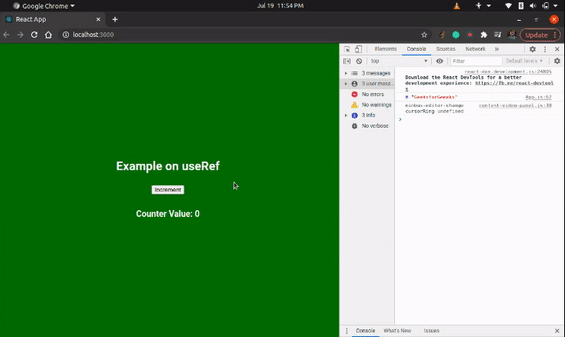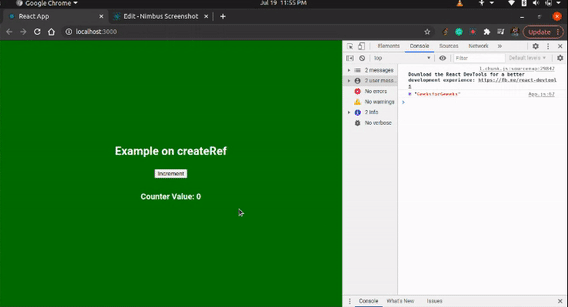Open In App

# Difference between useRef and createRef in ReactJS

What is a ref ?
A ref is defined as any value that does not trigger a component re-render when it is changed. This behavior is contrary to the function of states and props. A ref can be created in two ways- by the useRef hook or by the createRef function.

useRef: The useRef is a hook that uses the same ref throughout. It saves its value between re-renders in a functional component and doesn’t create a new instance of the ref for every re-render. It persists the existing ref between re-renders.

createRef: The createRef is a function that creates a new ref every time. Unlike the useRef, it does not save its value between re-renders, instead creates a new instance of the ref for every re-render. Thus implying that it does not persist the existing ref between re-renders.

Let’s look at an example to understand the differences more clearly.

Creating React Application:

• Step 1: Create a React application using the following command:

`npx create-react-app react-ref`
• Step 2: After creating your project folder i.e. react-ref, move to it using the following command:

`cd react-ref`

Project Structure:Example 1: In this example, we will create a ref by using useRef. In this case, we will notice that for every re-render our ref persists and thus we see the message we set inside the useEffect being repeated. Now write down the following code in the App.js file. Here, App is our default component where we have written our code.

## App.js

 `import React, { useEffect, useRef, useState } from ``"react"``;``import ``"./App.css"``;`` ` `export ``default` `function` `App() {``  ``const [counter, setCounter] = useState(0);``  ``const ref = useRef();`` ` `  ``useEffect(() => {``    ``ref.current = ``"GeeksforGeeeks"``;``  ``}, []);`` ` `  ``useEffect(``    ``() => {``      ``console.log(counter, ref.current);``    ``},``    ``[counter]``  ``);`` ` `  ``return` `(``    ``
``      ``
``        ``

Example on useRef

``        ````        ``
Counter Value: {counter}
{``" "``}``      ``
``    ``
``  ``);``}`

Step to Run Application: Run the application using the following command from the root directory of the project:

`npm start`

Output: Now open your browser and go to http://localhost:3000/, you will see the following output:Explanation: Since the useRef persists the ref value on re-renders, we can see the ref.current value on every re-render.

Example 2: In this example, we will create a ref by using createRef. In this case, we will notice that a new instance of ref is created after every re-render, thus we lose our message (GeeksforGeeks) after the initial display. Now write down the following code in the App.js file. Here, App is our default component where we have written our code.

## App.js

 `import React, { useEffect, createRef, useState } from ``"react"``;``import ``"./App.css"``;`` ` `export ``default` `function` `App() {``  ``const [counter, setCounter] = useState(0);``  ``const ref = createRef();`` ` `  ``useEffect(() => {``    ``ref.current = ``"GeeksforGeeeks"``;``  ``}, []);`` ` `  ``useEffect(``    ``() => {``      ``console.log(counter, ref.current);``    ``},``    ``[counter]``  ``);`` ` `  ``return` `(``    ``
``      ``
``        ``

Example on createRef

``        ````        ``
Counter Value: {counter}
{``" "``}``      ``
``    ``
``  ``);``}`

Step to Run Application: Run the application using the following command from the root directory of the project:

`npm start`

Output: Now open your browser and go to http://localhost:3000/, you will see the following output:Explanation: Since the createRef doesn’t persist the ref value on re-renders, we can see the ref.current value only once.

Difference between useRef and CreateRef: